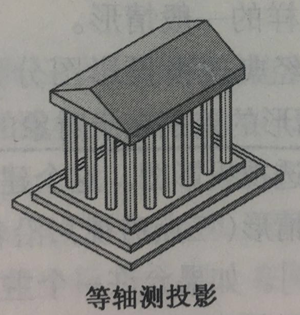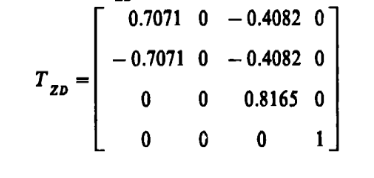# 背景图一图二

# 矩阵与坐标变换x=t
y=t^2
z=0

x = 0.7071 t - 0.7071 t^2
y = 0
z = -0.4082 t - 0.4082 t^2

x = 0.7071 t - 0.7071 t^2
y = 0
z = -0.4082 t - 0.4082 t^2

x=-i
z=(100-(i-10)
(i-10))/10（0<i<=20）

x=0.7071t
y=-0.4082t+0.8165(100-(t-10)(t-10))/10 （0<t<=20）

# 应用示例

z(x)=100 - (x/2-10)*(x/2-10))/2

x=t
z=100 - (t/2-10)*(t/2-10))/2

x = 0.7071t
z = -0.4082
t + 0.8165(100 - (t/2-10)(t/2-10))/2

x = 0.7071t-14.1422
z = -0.4082t + 0.8165(100 - (t/2-10)(t/2-10))/2+8.1642

(65,221) (65,199) (69,187) (72,176) (77,172) (81,176) (86,187) (90,205)

posted @ 2018-05-22 21:45  Gaoyb348  阅读(279)  评论(0编辑  收藏